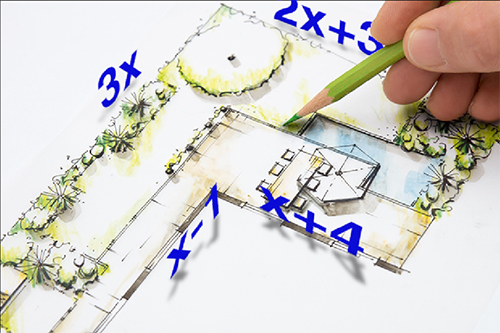• ### Browse All Lessons

##### Assign Lesson

Help Teaching subscribers can assign lessons to their students to review online!

 Tweet# Polynomials 05: Multiplying MonomialsIn this lesson we will begin to multiply polynomials and we will start with monomials, the simplest type. Multiplying polynomials can have many uses, one of which is to find the area of shapes which may change size but keep the same proportions.

Remembering that $y*y*y=y^3$, we can apply the same principle to $3x^2*5x$. If we break the equation apart we get $3*x*x*5*x$. We can then sort to get $3*5*x*x*x$ which simplifies to $15x^3$.

A more complex equation might look like $xy*4xy^2z*7x^4z^2*y^2z$.
This would expand to be $x*y*4*x*y*y*z*7*x*x*x*x*z*z*y*y*z$.
This would sort to be $4*7*x*x*x*x*x*x*y*y*y*y*y*z*z*z*z$ and simplify to be $28x^6y^5z^4$.

Of course you don't want to do all that work every time, but there is a simple pattern: Multiply the coefficients and add the exponents of like variables.

When multiplying a monomial by a polynomials we must multiply the monomial by each term in the polynomial. This means that $3x^2y(2x+6y^2)$ would be $6x^3y+18x^2y^3$. This principle applies regardless of the number of terms in the polynomial. $4ab^2c(2a^2+5bc^2-3c+b^2c)$ multiplies to be $8a^3b^2c+20ab^3c^3-12ab^2c^2+4ab^4c^2$.

Directions for this Lesson:
Watch the video below and complete the practice questions.

Required Video:

Related Worksheets:

Related Lessons: